# Bihar Board 12th Physics Objective Answers Chapter 13 Nuclei

Bihar Board 12th Physics Objective Questions and Answers

## Bihar Board 12th Physics Objective Answers Chapter 13 NucleiQuestion 1.
The natural boron of atomic weight 10.81 is found to have two isotopes 10B and nB. The ratio of abundance of isotopes of natural boron should be
(a) 11: 10
(b) 81 : 19
(c) 10:11
(d) 19 : 81
(d) 19 : 81

Question 2.
The mass number of iron nucleus is 56, the nuclear 1 density is
(a) 2.29 x 1016 kg m-3
(b) 2.29 x 1017 kg m-3
(c) 2.29 x 1018 kg m-3
(d) 2.29 x 1015 kg m-3
(b) 2.29 x 1017 kg m-3

Question 3.
Two nuclei have their mass numbers in the ratio of 1: 3. The ratio of their nuclear densities would be
(a) (3)1/3 : 1
(b) 1 : 1
(c) 1 : 3
(d) 3 : 1
(b) 1 : 1Question 4.
The radius of a spherical nucleus as measured by electron scattering is 3.6 fm. What is the mass number of the nucleus most likely to be?
(a) 27
(b) 40
(c) 56
(d) 120
(a) 27

Question 5.
The ratio of the nuclear radii of the gold isotope $$\begin{array}{l} 197 \\ 79 \end{array} \mathrm{Au}$$ and silver isotope $$\begin{array}{l} 107 \\ 47 \end{array} \mathrm{Ag}$$ is
(a) 1.23
(b) 0.216
(c) 2.13
(d) 3.46
(a) 1.23Question 6.
How much mass has to be converted into energy to produce electric power of 500 MW for one hour?
(a) 2 x 10-5 kg
(b) 1 x 10-5 kg
(c) 3 x 10-5 kg
(d) 4 x 10-5 kg
(a) 2 x 10-5 kg

Question 7.
If in a nuclear fusion reaction, mass defect is 0.3%, then energy released in fusion of 1 kg mass
(a) 27 x 1010 J
(b)27 x 1011 J
(c) 27 x 1012 J
(d) 27 x 1013 J
(d) 27 x 1013 J

Question 8.
The equivalent energy of 1 g of substance is
(a) 9 x 1013 J
(b) 6 x K)12 J
(c) 3 x 1013 J
(d) 6 x 1013 J
(a) 9 x 1013 JQuestion 9.
Fission of nuclei is possible because the binding energy per nucleon in them
(a) increases with mass number at low mass numbers
(b) decreases with mass number at low mass numbers
(c) increases with mass number at high mass numbers
(d) decreases with mass number at high mass numbers
(d) decreases with mass number at high mass numbers

Question 10.
Let mp be the mass of a proton, mn the mass of a neutron, M1 the mass of a $$\frac{20}{10} \mathrm{Ne}$$ Ne nucleus and M2 the mass of a $$\frac{40}{20} \mathrm{Ca}$$ nucleus. Then
(a) M2 = M1
(b) M2 > 2M1
(c) M2 < 2M1
(d) M1 < 10 (mn + mp)
(d) M1 < 10 (mn + mp)

Question 11.
The half life of $$\begin{array}{l} 238 \\ 92 \end{array} \mathrm{U}$$ undergoing a-decay is 4.5 x 109 years. The activity of 1 g sample of is
(a) 1.23 x 104 Bq
(b) 1.23 x 105 Bq
(c) 1.23 x 103 Bq
(d) 1.23 x 106 Bq
(a) 1.23 x 104 BqQuestion 12.
The half life of a radioactive substance is 20 s, the time taken for the sample to decay by 7/8th of its initial value is
(a) 20 s
(b) 40 s
(c) 60 s
(d) 80 s
(c) 60 s

Question 13.
The half life of a radioactive substance is 30 days What is the time takento disintegrate to 3/4th of its original mass?
(a) 30 days
(b) 15 days
(c) 60 days
(d) 90 days
(c) 60 days

Question 14.
The half life of polonium is 140 days. In what time will 15 g of polonium be disintegrated out of its initial mass of 16 g?
(a) 230 days
(b) 560 days
(c) 730 days
(d) 160 days
(b) 560 daysQuestion 15.
A 280 day old radioactive substance shows an activity of 6000 dps, 140 days later its activity becomes 3000 dps. What was its initial activity?
(a) 20000 dps
(b) 24000 dps
(c) 12000 dps
(d) 6000 dps
(b) 24000 dps

Question 16.
Tritium with a half-life of 12.5 years undergoing beta decay. What fraction of a sample of pure tritium will remain undecayed after 25 years.
(a) one half
(b) one fourth
(c) one third
(d) can’t say
(b) one fourth

Question 17.
The decay constant, for a given radioactive sample, is 0.3465 day-1. What percentage of this sample will get decayed in a period of 4 days?
(a) 100%
(b) 50%
(c) 75%
(d) 10%
(c) 75%Question 18.
Carbon dating is best suited for determining the age of fossils, if their age in years is of the order of
(a) 103
(b) 104
(c) 105
(d) 106
(b) 104

Question 19.
The half life of $$\begin{array}{l} 90 \\ 38 \end{array} \mathrm{Sr}$$ is 28 years. The disintegration rate of 15 mg of this isotope is of the order of
(a) 1011 Bq
(b) 1010 Bq
(c) 107 Bq
(d) 109 Bq
(b) 1010 Bq

Question 20.
A sample of radioactive material has mass m, decay constant λ, molecular weight M and Avogadro constant NA. The initial activity of the sample is
Am XmN A
$$(a) \lambda m (b) \frac{\lambda \mathrm{m}}{\mathrm{M}} (c) \frac{\lambda m N_{A}}{M} \quad (d) m N_{A} \lambda$$
$$(c) \frac{\lambda m N_{A}}{M} \quad$$Question 21.
Plutonium decays with half life of 24000 years. If plutonium is stored for 72000 years, the fraction of it that remains is
(a) 1/8
(b) 1/3
(c) 1/4
(d) 1/2
(a) 1/8

Question 22.
The number of beta particles emitted by a radioactive substance is twice the number of alpha particles emitted by it. The resulting daughter is an
(a) isomer of parent
(b) isotone of parent
(c) isotope of parent
(d) isobar of parent
(c) isotope of parent

Question 23.
Which of the following cannot be emitted by radioactive substances during their decay?
(a) Neutrinos
(b) Protons
(c) Electrons
(d) Helium nuclei
(b) ProtonsQuestion 24.
A radioactive decay can form an isotope of the original nucleus with the emission of particles
(a) one a and four β
(b) one a and two β
(c) one a and one β
(d) four a and one β
(b) one a and two β

Question 25.
Consider a and 3 particles and y-rays each having an energy of 0.5 MeV. In the increasing order of penetrating power, the radiation are respectively
(a) α,β,γ
(b) α,γ,β
(c) β,γ,α
(d) γ,β,α
(a) α,β,γ

Question 26.
An electron emitted in beta radiation originates from
(a) inner orbits of atom
(b) free electrons existing in the nuclei
(c) decay of a neutron in a nuclei
(d) photon escaping from the nucleus
(c) decay of a neutron in a nuclei

Question 27.
Complete the series $$^6 \mathrm{He} \rightarrow \mathrm{e}^{+6} \mathrm{Li}+$$
(a) neutrino
(b) antineutrino
(c) proton
(d) neutron
(a) neutrinoQuestion 28.
A nucleus of Ux1 has a half life of 24.1 days. How long a sample of Ux1 will take to change to 90% of Ux1.
(a) 80 days
(b) 40 days
(c) 20 days
(d) 10 days
(a) 80 days

Question 29.
An element A decays into an element C by a two step process A → B + 2He4 B → C + 2e. Then,
(a) A and C are isotopes
(b) A and C are isobars
(c) B and C are isotopes
(d) A and B are isobars
(a) A and C are isotopes

Question 30.
The equation $$4_{1}^{1} H^{+} \rightarrow_{2}^{4} H e^{2+}+2 e^{-}+26 M e V$$ represents
(a) β-decay
(b) γ-decay
(c) fusion
(d) fission
(c) fusionQuestion 31.
Light energy emitted by star is due to
(a) breaking of nuclei
(b) joining of nuclei
(c) burning of nuclei
(d) reflection of solar light
(b) joining of nuclei

Question 32.
Fast neutrons can easily be slowed down by
(a) the use of lead shielding
(b) passing them through water
(c) elastic collisions with heavy nuclei
(d) applying a strong electric field
(b) passing them through water

Question 33.
In the given reactions, which of the following nuclear fusion reaction is not possible?(a)Question 34.
If 200 MeV energy is released in the fission of a single nucleus of $$_{ 92 }^{ 235 }{ U }$$ the fission which are required to produce a power of 1 kW is
(a) 3,125 x 1013
(b) 1.52 x 106
(c) 3.125 x 1012
(d) 3.125 x 1014
(a) 3,125 x 1013

Question 35.
The gravitational force between a H-atom and another particle of mass m will be given by Newton’s law : $$\mathbf{F}=\mathbf{G} \frac{\mathbf{M} \cdot \mathbf{m}}{\mathbf{r}^{2}}$$ is in km and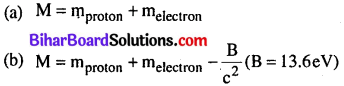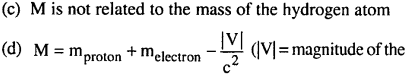(b)

Question 36.
When a nucleus in an atom undergoes a radioactive decay, the electronic energy levies of the atom
(a) do not change for any type of radioactivity
(b) change for a and P radioactivity but not for y- radioactivity
(c) change for a-radioactivity but not for others
(d) change for P-radioactivity but not for others.
(b) change for a and P radioactivity but not for y- radioactivityHints & Explanations

Question 1.
(d) Let abundance of B10 be x%
Abundance of B11 = (100 – x)%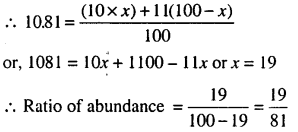Question 3.
(b) A1 : A2 = 1 : 3
Their radii will be in the ratio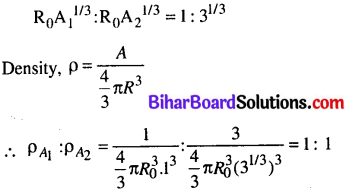Their nuclear densities will be the same

Question 4.
(a) Nuclear radius, R = R0(A)1/3, where A is the mass
number of a nucleus
Given, R = 3.6 fm
∴ 3.6fm = (1.2fm)(A1/3) (∴ R0 = 1.2fm)
or, A = (3)3 = 27Question 5.
(a) Here, A1 = 197 and A2 = 107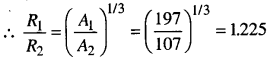Question 6.
(a) Here, P = 500 MW = 5 x 108 W,t = 1 h = 3600 s
Energy produced, E = P x / = 5 x 108 x 3600
= 18 x 1011 J
As E = mc2Question 7.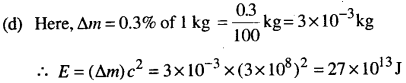Question 8.Question 11.
(a) T1/2 = 4.5 x 109 years
= 4.5 x 109 x 3.16 x 107 : 1.42 x 1017 s
One kmol of any isotope contains Avogadro’ number of atoms, so 1 g of $$_{ 92 }^{ 238 }{ U }$$ containsQuestion 12.
(c) After three half lives, the fraction of undecayed nuclei $$=\left(\frac{1}{2}\right)^{3}=\frac{1}{8}$$
∴ Time taken for the sample to decay by (1 – 1/8)th or $$\frac{7^{t h}}{8}$$ of initial value. = 3T1/2 = 3 x 20 = 60 s

Question 13.
(c) Here, $$\frac{N_{0}-N}{N_{0}}=\frac{3}{4} \text { or } \frac{N}{N_{0}}=\frac{1}{4}=\left(\frac{1}{2}\right)^{2}$$
∴ No of half lives, n = 2
Time taken to disintegrate
= Half life x No. of half lives
= 30 x 2= 60 days

Question 14.Question 17.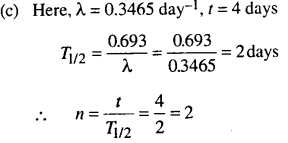Hence, sample left undecayed after a period of 4 days.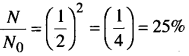∴ Sample decayed = 75%

Question 18.
(b) Carbon dating is best suited for determining the age
of fossils, if their age is of the order of 104 years.

Question 19.
(b) Here, T1/2 = 28 years = 28 x 3.154 x 107 sQuestion 28.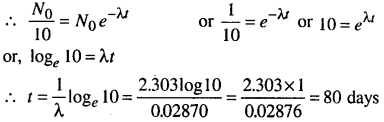Question 34.
Let the number of fissions per second be n. Energy released per second
= n x 200 MeV = n x 200 x 1.6 x 10~13 J

Energy required per second = power x time = 1 kW x Is = 1000J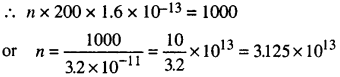error: Content is protected !!